• Testimonial
• Web Stories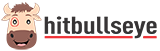## Learning Home## Not Now! Will rate later## Arithmetic Reasoning: Solved Examples• In a college, there are 34 girls who have car. If these constitute one fifth of the girls and the total no. of girls is two-fifth of the total no. of students in the college, what is the no. of boys in the college? A. 425 B. 220 C. 185 D. 255 Answer: Option D Let the number of girls be x. So, (1/5) x = 34 or x = 34 x (5/1) = 170. If total no. of students is y, then (2/5) y = 170 or y = 170 x (5/2) = 425. Therefore, Number of boys in the college = (425 - 170) = 255
• Joseph is now 1/4 th times as old as his mother Ketty. Four years hence, his mother will be three times as old as her son. The age of his mother Ketty (in years) is A. 32 B. 35 C. 8 D. 24 Suggested Action FREE Live Master Classes by our Star Faculty with 20+ years of experience. Register Now Answer: Option A Let Joseph’s mother Ketty age be 4x years. Then, Joseph’s age = x years. Four years hence, Joseph's age = (x + 4) years and Joseph’s mother Ketty age = (4x + 4) years. So, 4x+4= 3 (x+4) 4x-3x= 12-4; x = 8. Joseph's mother Ketty age = 4x= 4 x 8=32 years
• A director's total salary is sum of his rent charges, deposits and expenditure. During one month his deposits is equal to expenditure which is 2/3 of rental charges. What fraction of his total income saves in deposits? A. 4/9 B. 5/4 C. 5/8 D. 2/7 Answer: Option D Let Rent charges= Rs.x, Deposits = Expenditure= Rs. 2/3 x Salary =Rental Charges+ Deposits + Expenditure             = x + (2/3)x + (2/3)x = (7/3)x Required Fraction = (2x / 3) x (3/7x) = 2/7
• Arithmetic Reasoning Concepts & Basics
• Practice Questions Level 01
• Arithmetic Reasoning Solved Examples
• Practice Questions Level 02
• Rina & Rohit are playing a game, Rina writes down all the natural numbers from 1 to 100, then Rohit has to calculate how many times does she write four? A. 11 B. 18 C. 20 D. 21 Answer: Option C From 1 to 100, there are exactly 10 numbers with 4 as their tens digit – 40, 41, 42 ,43 ,44, 45,46,47,48,49 ; and 10 numbers with 4 as their unit digit: 4,14,24,34,44, 54,64,74,84,94 So, the required number of numbers = 10 + 10 = 20
• At Anoop’s Birthday party, there are exactly 57 persons including Anoop. They shake hand with each other.  Find the total number of handshakes. A. 1956 B. 1599 C. 1965 D. 1596 Answer: Option D 1st person shakes hand with 56 persons, 2nd person shakes hand with 55 persons because he already shakes hand with the 1st person. And 3rd person shakes hand with 54 and so on. Therefore, total number of  handshakes = 56+55+54+…….+3+2+1 = 1+2+3+…………….+54+55+56 Formula for sum of Natural numbers= n(n+1) / 2 Total number of  hand shakes = Sum of 56 natural numbers= 56(56+1) / 2 = 56x57/2 = 1596
• Four tubelights begin to light together and light at intervals of 5, 8, 9 and 11 seconds respectively. How many times will they light together in two hours including the one at the start? A. 2 times B. 3 times C. 4 times D. 5 times Answer: Option A L.C.M. of 5, 8, 9 & 11 is 3960. So, the tubelights will light together after every 3960 seconds i.e. 1 hour 6 minutes. Therefore, in two-hours (or 120 minutes), the tubelights will light together 2 times, including the one at the start.
• An airplane starts from country X. The no. of males in the airplane is thrice of the no. of females. In country Y, six females enter and 10 males leave the airplane at country W. Now, no. of males & females is equal. How many persons entered in the airplane from the beginning? A. 15 B. 30 C. 36 D. 32 Answer: Option D Originally, let number of females = x. Then, number of males = 3x. So, in city Y, we have: (3x - 10) = (x + 6) or x =8. Therefore Total number of persons in the beginning = (x + 3x) = 4x = 32
• There are four friends Amit, Ram, Rohit and Kirti. In an examination, the sum of their marks is 450. Rohit’s Marks is equal to the twice the sum of Ram and Kirti’s marks. Amit marks are equal to Rohit’s marks.The person who gets 95 marks and above it will go to next level. Who are the people which go to next level examination? A. Amit & Rohit B. Rohit & Ram C. Ram & Kirti D. Kirti & Rohit Answer: Option A Let a, b, c, and d is the marks of Amit, Ram, Rohit and Kirti respectively. Clearly, we have : a+b+c+d=450….(i) c=2(b+d) ….(ii) a=c….(iii) from i & ii a+c+c/2 =450; (we have a=c) c+c+c/2= 450 5/2c=450 c=180; a=180 b+d= c/2= 180/2=90 a, c is more than 95, b, d is not more than 90. Therefore, Amit and Rohit will go to next level examination.
• State authority decided to make fence around the square garden of 400×400 m 2 . They decided to put poles at a distance of 10m. How many poles did they need altogether? A. 100 B. 156 C. 108 D. None of these Answer: Option B Since, 38 poles on the edge of garden and one pole at the corners of garden. Total number of poles needed = 38 x 4 + 4 = 152+4 = 156.
• In a state, 70% of the girls are live in urban area while 35% of boys are live in rural areas. If the ratio of the girls to that of the boys is 5: 3, then what percent of the total population is live in rural area? A. 33% B. 30.78% C. 50% D. 31.875% Answer: Option D Let the number of girls and boys be 5x and 3x respectively. Then, Rural Population = (100-70) % of 5x+35% of 3x = (30/100) x (5x) + (35/100) x (3x) = (51/20)x Required % = (51x / 20) x (1 / 8x) x 100 = 31.875%## Most Popular Articles - PS## Syllogism Practice Questions : Level 01## Syllogisms : Concepts and Theory## Syllogism Practice Questions : Level 02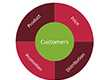## Venn Diagram Approach## Syllogisms : Solved Examples## Finding Missing Character Practice Questions : Level 01## Finding the missing character: Concepts & tricks## Data Sufficiency Reasoning: Concepts & Tricks## Analogies : Concepts & Basics## Analogies : Solved Examples

• Learn on-the-go
• Unlimited Prep Resources
• Better Learning Experience
• Personalized Guidance

Get More Out of Your Exam Preparation - Try Our App!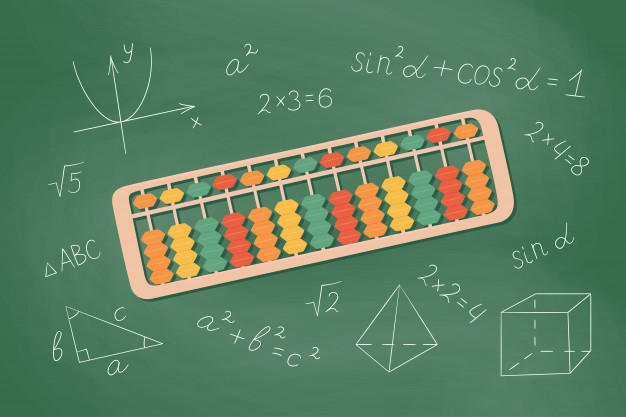## A 2023 Complete ASVAB Arithmetic Reasoning Study Guide

The ASVAB Arithmetic Reasoning test measures a candidate’s ability to solve problems representing word problems and delivers mathematical questions and equations. These may not only be simple questions involving addition, subtraction, multiplication, or division but may also require reasoning skills to determine what is really being asked for and finding the best answer.

January 1, 2022

The ASVAB Arithmetic Reasoning test evaluates a candidate’s ability to solve word problems and provides mathematical questions and equations. These questions may include not only simple addition, subtraction, multiplication, or division, but also reasoning skills to determine what is truly being asked for and the best answer.

The computerized version of the CAT-ASVAB has 15 questions and takes 39 minutes to complete; the paper-and-pencil version has 30 questions and takes 36 minutes to complete. In addition to this ASVAB Arithmetic Reasoning study guide, we offer hundreds of ASVAB practice questions and ASVAB practice test 2023 to help you familiarize yourself with the test format. Let’s give it a try!

## ASVAB Arithmetic Reasoning Concepts

The following arithmetic concepts are included in your exam:

• Arithmetic : This concentrates on basic arithmetic such as addition, subtraction, division, and multiplication.
• Percentages :  This kind of question relates to calculating cost price, sale price, discount, etc.
• Ratio and proportion : Ratio/proportion-related questions are solved by using simple formulas.
• Interest :  Interest-related questions may require more complicated formulas.
• Numbers : This asks for your knowledge of whole numbers, decimals, real numbers, fractions, imaginary numbers, etc.

The Arithmetic Reasoning section is used to calculate your  Armed Forces Qualification Test  ( AFQT ) score so you should get a good score on this section. Take the  Arithmetic Reasoning ASVAB practice test  as much as possible to familiarize yourself with the test format and determine clearly your strength and weakness.

These word problems may have some technical terms, besides basic terms, such as area, perimeter, integer, or ratio, which are expected to be common mathematical knowledge. When solving Arithmetic Reasoning questions, you must pay attention not only to the numbers mentioned in the problem but also to the wording, the format of the paragraph, buzzwords, and more.

## ASVAB Arithmetic Reasoning Tips and Tricks

Finding “buzzwords”.

These words or phrases of emphasis indicate the action you will need to solve the problem. For example, if a problem mentions “difference”, “fewer” or “take away”, it may require you to use subtraction, while some words like “times”, “product” or “double” may stand for multiplication. Before solving the problems, make sure you carefully read and identify what process it requires. It will show you the direction you should take to solve the overall equation.

## Identify numbers

Word problems can be simple with an addition or subtraction of 2 numbers, or they can include more complex numbers and operations. Pay attention to all the given numbers and figures within the body of the paragraph. Read carefully these numbers, and then determine which of the numbers are relevant to solve the problem and which of them are misleading you.

Make sure you perform them in the right order. 6 – 8 and 8 – 6 bring two very different results and may affect your pass or fail. Be as careful as possible with the number to avoid unnecessary mistakes.

## Paragraph Format

When dealing with the Arithmetic Reasoning section, you should notice that many word problems may contain irrelevant information that is used as a filler to distract you from the real question being asked. You must learn to scan all over the problem, disregard this misleading verbiage and focus on the portions that will help you answer the problem. Just because something is included in a paragraph doesn’t mean that it is important and must be used.

By identifying the format and context of the paragraph combined with the buzzwords and numbers, you can build a completed, simplified equation. Be sure that you select all the necessary information, make a proper equation, and solve it.

If you run into a problem that stumps you, skip it to move ahead to another one and then come back to it if you have time. Do not waste too much time on a problem, try to quickly solve the other questions that you are certain about it.

## Steps to solving ASVAB word problems

Here is the suggested route to answer the questions in the ASVAB Arithmetic Reasoning test.

Because of the limited time, you may push yourself to solve a problem quickly. This easily leads to the disaster of failing the test. Word problems can be tricky, so you have to thoroughly read each to identify exactly what is being asked for.

## Determine the method used to answer

After thoroughly understanding the problem, you’ll need to gather all the relevant data from the problem and decide what is the best way to solve the question it is asking.

## Setup the equations

Once you have determined the method used to answer, you need to set all the relevant data into an equation that will lead you to the correct answer.

## Solve equations and review results

When you have the equations for the question, solve it to find the final result. Then quickly review to make sure there is no regretful mistake in the solving progress.

## Basic Arithmetic Review for ASVAB Exams

Before starting to practice the Arithmetic problems, let’s review all the basic definitions, properties, and Arithmetic Reasoning formulas you may need in the ASVAB Arithmetic.

## Types of Numbers

Natural number.

Natural numbers (i.e. counting numbers) are numbers that are used for counting and ordering. They can be expressed mathematically as {1, 2, 3, 4, 5, …}

## Even Number

Even numbers are natural numbers that are divisible by 2.

2ℕ = { 2, 4, 6, 8, 10, 12, 14, … }

Odd numbers are natural numbers that are not divisible by 2.

2ℕ + 1 = { 1, 3, 5, 7, 9, 11, 13, 15…}

## Prime Number

A prime number is a number greater than 1 that is only divisible by 1 and by itself.

2, 3, 7, and 11 are prime numbers

P = { 2, 3, 5, 7, 11, 13, 17, 19,…}

## Composite Number

Composite numbers are the product of some prime numbers. For example:

8 = 2 ⋅ 2⋅ 2

## Whole Number

In mathematics, whole numbers are the basic counting numbers 0, 1, 2, 3, 4, 5, 6, … and so on.

An integer number includes all positive whole numbers (a positive integer), and negative whole numbers (a negative integer), or zero.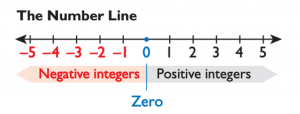We can put that all together like this:

Integers = { …, −4, −3, −2, −1, 0, 1, 2, 3, 4, … }

## Fraction/Rational Numbers

A fraction/rational number is a ratio of two integer numbers in the form of A/B , where A and B are integers and B#0 .

A is called Numerator

B is called Denominator

## Irrational Numbers

Real numbers that cannot be written as the quotient of two

integers but can be represented on the number line.

-2√3 , √2, π

## Real Numbers

Include all numbers that can be represented on the number line, that is, all rational and irrational numbers.

## The Basic Number Properties

Four basic properties of numbers include commutative, associative, distributive, and identity. You should familiarize yourself with each of these before taking the Arithmetic Reasoning subtest.

Identity Property of Zero :

• Inverse Property :

a + (-a) = 0

• Commutative Property :

When adding two numbers together, the outcome (sum) is the same regardless of the order the numbers are placed in.

a + b = b + a

For example, the two following equations end up with the same result:

4 + 6 = 10 or 6 + 4 = 10

Associative Property :

When adding multiple numbers together, the outcome (sum) is the same regardless of the order the numbers are placed in.

(a + b) + c = a + (b + c)

## Properties of subtractions

Non-Commutativity

Unlike addition, the order of two numbers in subtraction changes all the results. In other words, the subtrahend and minuend are distinct factors when subtracting and they cannot be switched order-wise (except subtrahend and minuend are equal).

a – b # b – a

For example :

8 – 6 = 2 is not the same as 6 – 8 = -2

Non-Associativity

When subtracting multiple numbers, the order of the numbers does matter. Subtracting numbers in different orders will result in different outcomes.

## Properties of multiplication

Property of Zero :

Identity Property of One :

a × 1 = a , where a # 0

a × 1/a  = 1 , where a # 0

When multiplying two numbers together, the product is the same regardless of the order in the numbers are placed in.

a × b = b × a

2 × 3 = 6 or 3 × 2 = 6

Associative Property :

When multiplying multiple numbers together, the product is the same regardless of the order in the numbers are placed in.

(a × b) × c = a × (b × c)

(2 × 3) × 4 = 2 × (3 × 4) = 24

## Properties of division

0/a = 0 , when a # 0 .

Property of One :

a/a = 1 when a # 0 .

a/1 = a × 1 .

## Absolute Value

The absolute value of a number is always greater than 0.

If a > 0, |a| = a.

If a < 0, |a| = a.

For example, |8| = 8 and |-8| = 8. In each case, the answer is positive.

## Order of Operations

Step 1 : Parentheses – Simplify any expressions inside parentheses.

Step 2 : Exponents (Powers, Roots) – Work out any exponents.

Step 3 : Multiply or Divide before you Add or Subtract

Step 4 : Addition and Subtraction These are done last, working from left to right.

For example:

10 – 8 × 4 + (6 ÷ 3) + 5 × 2 3

= 10 – 8 × 4 + 2 + 5 × 8

= 10 – 32 + 2 + 40

>> More: General Science ASVAB Study Guide

## Adding and subtracting with negatives

– a – b = (-a) + (-b)

– a + b = b – a

a – (-b) = a + b

– 2 – 3 = (-2) + (-3) = -5

– 2 + 5 = 5 – 2 = 3

2 – (-3) = 2 + 3 = 5

## Multiplying and dividing with negatives

-a × b = -ab

-a × -b = ab

(-a)/(-b) = a/b, b # 0

(-a)/b = -a/b, b # 0

-2 × 3 = -6

-2 × -3 = 6

(-2)/(-3) = ⅔

(-2)/3 = -⅔

Fractions are another way to express division. The top number of a fraction is called the numerator, and the bottom number is called the denominator.

## Least common multiple

The LCM of a set of numbers is the smallest number that is a multiple of all the given numbers. For example, the LCM of 5 and 6 is 30, since 5 and 6 have no factors in common.## Greatest common factor

The GCF of a set of numbers is the largest number that can be evenly divided into each of the given numbers. For example, the GCF of 24 and 27 is 3, since both 24 and 27 are divisible by 3, but they are not both divisible by any numbers larger than 3.

Fractions must have the same denominator before they can be added or subtracted.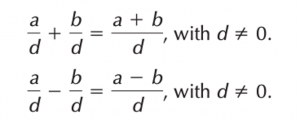If the fractions have different denominators, rewrite them as equivalent fractions with a common denominator. Then add or subtract the numerators, keeping the denominators the same. For example :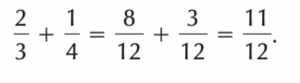## Multiplying and dividing fractions

When multiplying and dividing fractions, a common denominator is not needed. To multiply, take the product of the numerators and the product of the denominators :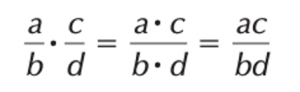⅔ × ⅛ = (2 × 1 )/(3 × 8) = 2/24 = 1/12

To divide fractions, invert the second fraction and then multiply the numerators and denominators :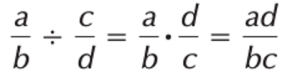⅔ ÷ ⅛ = (2 × 8)/(3 × 1) = 16/3.

Read more >> ASVAB Math Study Guide

## What is in the ASVAB Arithmetic Reasoning section?

Your ability to solve arithmetic and math word problems will be tested on the Arithmetic Reasoning subtest. On the computer test, you will be given 15 scored questions and it’s 30 scored questions on the paper and pencil test, but you might also be given tryout questions.

## How can you improve your arithmetic reasoning skills for ASVAB?

Taking ASVAB practice tests like the ones provided above is one of the best ways to prepare for a high score on Arithmetic Reasoning. These ASVAB practice questions will allow you to answer questions similar to those on the test in a timed environment that is quite similar to the actual test experience.

Hope that the above ASVAB Arithmetic Reasoning study guide helps you grasp all the important information you need for your coming exam!

We hope you find our ASVAB Study Guide 2023 useful in learning everything you need to know for your upcoming exam!Marvin Shaw is a homeschooling high school teacher and an ASVAB tutor. He's one of the creators of our ASVAB question collection. With his broad knowledge and a big love for the U.S. military, he's mentored hundreds of students to successfully enter the military.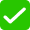Fighting the spread of false information on the Internet is a top priority to me. All of my content is edited by a professional and fact checked by an external fact-checking service.

Fact-checking is a procedure that verifies information in order to ensure the accuracy and authenticity of reporting. Fact-checking can be undertaken before or after the publication. Internal fact-checking is performed in-house by the publisher; external fact-checking is performed by a third party.## ASVAB General Science Study Guide In 2023

April 25, 2023## A Complete Pictorial History Of US Navy Uniforms From 1776 To 1967

Follow this article to find out the pictorial history of US Navy uniforms from 1776 to 1976 and beneficial information about US Navy uniforms as well!

March 9, 2022## Can An Immigrant Join The Army In The United States? [2023 Updated]

Can an immigrant join the Army? You do not have to be a U.S. citizen to enlist in the Army but you need to meet some requirements. Read this post to know more.

March 5, 2022

ASVAB Tutor: Home / ASVAB Study Guides / ASVAB Arithmetic Reasoning Study Guide

## ASVAB Arithmetic Reasoning Study Guide

Arithmetic reasoning refers to the process of solving math word problems – you know those questions you had in elementary, middle and high school that might involve two trains traveling at different speeds or determining how many different pieces of fruit Tommy brought home from the grocery store.

Whether you enjoy these types of problems or dread them, there is a process you can use to make solving them quicker and easier.

And it is important that you answer as many of these questions correctly as you can – the Arithmetic Reasoning subtest of the Armed Services Vocational Aptitude Battery is part of the Armed Forces Qualification Test (AFQT) score which determines your eligibility for military service.  Your Line Score is a composite score that the various military branches use to determine what jobs you qualify for.

Your test administrator will provide you with scratch paper and a number two pencil for this portion of the ASVAB. Calculators are not allowed.

If you are taking the pencil-and-paper test, you will 36 minutes to answer 30 questions and if you are taking the computer version, you will have 39 minutes to answer 16 questions.

## The Content

The Arithmetic Reasoning Subtest is composed of math word problems. That means you must pay attention not only to the numbers in the problem but also the wording, the paragraph format, buzzwords and more.

Keep in mind this subtest is called Arithmetic Reasoning for a reason – you will have to solve a math problem using addition, subtraction, multiplication or division but you will also have to use reasoning skills to figure what is really being asked for and the best way to obtain that answer.

Here are the steps to answering the questions in the Arithmetic Reasoning Subtest correctly.

During a timed test, our inclination is to rush through each problem afraid that we’ll run out of time. Doing that in this particular part of the exam can be a recipe for disaster. Word problems can be tricky, so you have to carefully read each to discern exactly what is being asked for.

• Determine the question

After reading the problem, the next step is to determine exactly what the question is asking.

• Determine how to answer that question

In this step, you’ll need to look back at the problem and determine the best way to answer the question it is asking. This step will involve extracting the relevant facts from the problem.

• Create an equation or equations

Once you have determined the best way to provide the answer and have gathered the relevant facts, you need to put those facts into an equation that will give you the correct answer.

• Solve and review

In this final step, you will solve your equation or equations, do a quick review to make sure you have arrived at an answer that satisfies what the question is looking for and then record your answer.

Look for “buzzwords”

These words or phrases of emphasis can let you know what type of equation you will need to solve the problem. For example, if a problem mentions “less than” or “fewer” or “minus” there’s a good chance you may need to use subtraction and if it uses words like “more than” or “more” or “add” you may need to use addition. Just be sure to carefully read the problem and very often the wording of the problem itself will clue you in on the direction you need to take.

Dealing With Numbers

Word problems can be simple, in which you only need to add two numbers together, or they can be complex involving numerous numbers and numerous operations. The key to solving either type of question is to pay close attention to the numbers. Be sure to keep them in the right order and to use them in the correct manner. Remember, rushing through a problem can lead to unnecessary mistakes. You want to be extra careful with the numbers.

Paragraph Format

Many word problems may contain extra verbiage that serves no real purpose other than to distract you from the real question being asked. You must learn to disregard this extra information and focus in on the facts that will get you the answer to the problem. Don’t be afraid to “filter out” unnecessary information. Just because something is contained in a word problem doesn’t mean that it is important and must be considered.

## Preparing to Ace The Arithmetic Reasoning Section of the ASVAB Test

One of the best things you can do to prepare to score high on Arithmetic Reasoning is take practice tests like those offered  here.

These practice questions will give you the opportunity to answer questions similar to what will be on the test in a timed atmosphere that is also similar to the actual test experience.

Additional techniques you may want to consider using to boost your score include:

• Remember after reading the problem to disregard unessential information and focus in on only the important facts
• If you run into a problem that stumps you, skip it and then come back to it if you have time. Because this is a timed test, it’s better for you to answer the questions you can quickly and then work back to the ones that are more difficult to make better use of the available time.
• Stay calm. More than likely you are going to run into a problem or problems that are difficult. You can’t let this throw you off your game. Letting a question take too much of the available time or letting it affect your approach to subsequent questions can lead to a poor score.

Arithmetic Reasoning is an important part of the ASVAB both in regards to your AFQT score and what jobs you qualify for – so be sure to study math word problems extensively before taking the test. Take our practice test now and see where you stand – you may already be a wiz at solving these problems or you may need more practice … our practice test can help you determine where you stand.

## ASVAB Study Guides

Using the right ASVAB study guide is essential to ensure the best possible outcome when preparing for the exam.

## ASVAB Arithmetic Reasoning Practice Test

The ASVAB Arithmetic Reasoning (AR) test evaluates your ability to solve arithmetic word problems. To help you prepare for this section of the ASVAB, this page contains everything you need to know, including what topics are covered, how many questions there are, and how you can study effectively.

Click “Start Test” below to take a free ASVAB Arithmetic Reasoning practice test!## What’s on the ASVAB Arithmetic Reasoning Test?

The computerized version of the Arithmetic Reasoning test (CAT-ASVAB) contains 15 questions and has a time limit of 55 minutes, while the paper-and-pencil version (P&P-ASVAB) contains 30 questions and has a time limit of 36 minutes.

## ASVAB Online Prep Course

If you want to be fully prepared, Mometrix offers an online ASVAB Prep Course. The course is designed to provide you with any and every resource you might want while studying. The ASVAB Course includes:

• In-Depth Lessons Covering Every Topic
• 1,550+ ASVAB Practice Questions
• Over 240 Video Tutorials
• More than 300 Electronic Flashcards
• Money-back Guarantee
• Free Mobile Access

The ASVAB Prep Course is designed to help any learner get everything they need to prepare for their ASVAB exam. Click below to check it out!

## How many Arithmetic Reasoning questions are on the ASVAB?

There are 15 questions on the CAT-ASVAB, and there are 30 questions on the P&P-ASVAB.

## What is the time limit for the Arithmetic Reasoning test on the ASVAB?

The CAT-ASVAB allows you 55 minutes to finish the Arithmetic Reasoning test, while the P&P-ASVAB allows you 36 minutes.

## How can I improve arithmetic reasoning skills for the ASVAB?

Knowing how to approach arithmetic reasoning questions will help you tackle them on the test. Try taking a practice test and following these steps:

• Deconstruct the question you are being asked to identify exactly what you are being asked.
• Rewrite the question as a mathematical equation.
• Make a list of the information/formulas you will need to remember to solve the problem.
• Write down each step you need to take to solve the problem so you can have an easy reference point.

## How can I pass the Arithmetic Reasoning test on the ASVAB?

A passing score on the Arithmetic Reasoning test will differ depending on which branch of service you are trying to enlist in and which job you applying for.

To ensure you’re prepared, check out the Mometrix ASVAB study guide:By Peter Rench

Peter Rench joined Mometrix in 2009 and serves as Vice President of Product Development, responsible for overseeing all new product development and quality improvements. Mr. Rench, a National Merit Scholar, graduated magna cum laude with a Bachelor of Science in Mechanical Engineering and a minor in mathematics from Texas A&M University.

ASVAB Practice Test Home

by Mometrix Test Preparation | This Page Last Updated: September 13, 2023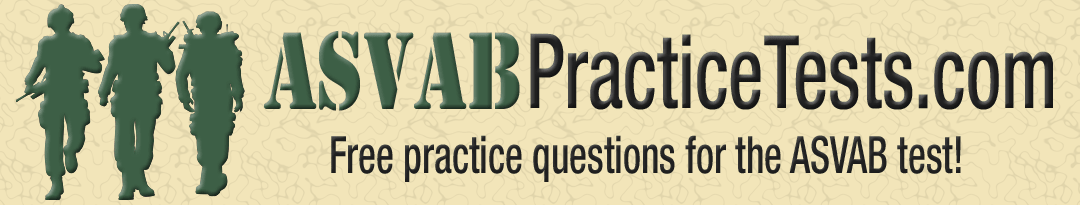## ASVAB Arithmetic Reasoning Practice Test

ASVAB Math Test Prep

Congratulations - you have completed .

You scored %%SCORE%% out of %%TOTAL%%.

Your performance has been rated as %%RATING%%

## There are 5 blue marbles, 4 red marbles, and 3 yellow marbles in a box. If Jim randomly selects a marble from the box, what is the probability of selecting a red or yellow marble?

If kayla left a \\$10.47 tip on a breakfast that cost \\$87.25, what percentage was the tip, a phone company charges \\$2 for the first five minutes of a phone call and 30 cents per minute thereafter. if malik makes a phone call that lasts 25 minutes, what will be the total cost of the phone call, if 10 inches on a map represents an actual distance of 100 feet, then what actual distance does 25 inches on the map represent, five years ago, amy was three times as old as mike. if mike is 10 years old now, how old is amy.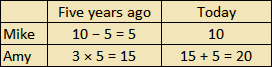## At a used book store, Valentina purchased three books for \\$2.65 each. If she paid with a \\$20 bill, how much change did she receive?

Mia earns \\$8.10 per hour and worked 40 hours. charlotte earns \\$10.80 per hour. how many hours would charlotte need to work to equal mia’s earnings over 40 hours, aisha wants to paint the walls of a room. she knows that each can of paint contains one gallon. a half gallon will completely cover a 55 square feet of wall. each of the four walls of the room is 10 feet high. two of the walls are 10 feet wide and two of the walls are 15 feet wide. how many 1-gallon buckets of paint does aisha need to buy in order to fully paint the room, there are 4 red marbles and 8 green marbles in a box. if emma randomly selects a marble from the box, what is the probability of her selecting a red marble.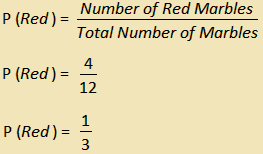## Oscar purchased a new hat that was on sale for \\$5.06. The original price was \\$9.20. What percentage discount was the sale price?

There are two pizza ovens in a restaurant. oven #1 burns three times as many pizzas as oven #2. if the restaurant had a total of 12 burnt pizzas on saturday, how many pizzas did oven #2 burn, sofía is driving to texas. she travels at 70 kilometers per hour for 2 hours, and 63 kilometers per hour for 5 hours. over the 7 hour time period what was sofía’s average speed, stephen signed up to bring 5 gallons of lemonade to the company picnic. he has a 5-gallon bucket which contains 3.5 gallons of lemonade. how many pints of lemonade will he need to add in order to fill the bucket, if a car travels 360 kilometers in 5 hours, how many kilometers will it travel in 9 hours when driving at the same speed, maya purchased a boat for \\$18,340. its value depreciated by 15% in the first year she owned it. what was her boat worth at the end of this first year, the radar system beeps once every second. how many times will it beep in 3 days.

Having trouble with the practice test?

Try our recommended ASVAB Math Prep Course!   START NOW## ASVAB Arithmetic Reasoning Math Study Guide

• 1.  What is the ASVAB Arithmetic Reasoning Section?
• 2.  How Many Questions are in the ASVAB Arithmetic Reasoning Section?
• 3.   What Topics are on the ASVAB Arithmetic Reasoning?
• 4.  How to Tackle ASVAB Math Word Problems (3 Step Strategy + Video)
• 5.   ASVAB Arithmetic Reasoning Practice Questions (with Video Solutions)
• 6.   ASVAB Arithmetic Reasoning Math Formulas (Quizlet)
• 7.  Take a Free ASVAB Arithmetic Reasoning Practice Test (Online and Printable)
• 8.  Do You Feel Like You Can’t Pass the ASVAB Math Sections? Don’t Worry; We’ve Got Your Back.
• 9.   How Do You Raise Your Arithmetic Reasoning Score? (Tips)
• 10.  Conclusion - What Should You Do Next?

## 1. What is the ASVAB Arithmetic Reasoning Section?

2. how many questions are in the asvab arithmetic reasoning section, 3. what topics are on the asvab arithmetic reasoning.

• General Word Problems - Includes word problems that don't have a specific formula. You will have to understand the overall situation and create a solution.
• Ratios and Proportions - Includes word problems that compare the same things in the same way.
• Distance, Rate, and Time - Includes word problems involving the Distance, Rate, and Time formula (Distance = Rate x Time)
• Percentages  - Includes word problems involving basic percent calculations, tax, discount, simple interest, and compound interest.
• Fractions - Any type of word problem that includes fractions and fraction operations (addition, subtraction, multiplication, division)
• Geometry (Perimeter, Area, Volume) - Includes word problems involving measurement. You'll need to know your formulas for perimeter, area, volume, and surface area of different shapes.
• Unit Conversion - Includes word problems that require you to change units to properly complete it. Harder questions include converting rate units (example: turning meters per second to kilometers per hour)
• Statistics (Mean, Median, Mode) - Includes word problems involving basic statistics. Harder questions involve working backwards, such as finding one of the numbers in a data set when given the mean.
• Probability - Includes word problems involving simple probability, compound probability, and the fundamental counting principle (knowing how many different ways you can arrange a set of items).

## 4. How to Tackle ASVAB Math Word Problems (3 Step Strategy + Video)

• The first thing you should always do when tackling any word problem is understand what the problem is asking you to figure out! Do not read the whole problem .
• Why is this the first step?   Think about it like this: Do you like playing a game if you don't know how to win? Do you ever get in your car and drive without knowing where you're going first?
• You might think this just means "writing down the numbers." Nope. That's 1% of the work. Whenever you write a number down,  always make sure to write down what the number means. That's going to help you big time when you're making your plan.
• Remember: the numbers can change, but the process won't. So instead of writing down "45", make sure to write down "45 miles per hour".
• This is where you connect  what you want  and  what you have. If you wrote down the numbers and what they mean , you will be able to see a clear path to the answer.
• What if you don't see a clear path? Then you have to ask yourself "How does this help me get what I need?

## 5. ASVAB Arithmetic Reasoning Practice Questions (with Video Solutions)

• General (Arithmetic Operation) Word Problems

## Proportion Word Problems

Distance, rate, and time word problems.

• Percent Word Problems
• Fraction Word Problems
• Geometry Word Problems (Area, Perimeter, and Volume)

## Unit Conversion Word Problems

• Mean, Median, and Mode Word Problems

## Probability Word Problems

General (arithmetic operations) word problems, percent word problems , fraction word problems , geometry: perimeter, area, and volume word problems, mean, median, mode word problems, 6. asvab arithmetic reasoning math formulas (quizlet sets), asvab arithmetic reasoning quizlet set: formulas, asvab unit conversions quizlet set:, 7. take a free asvab arithmetic reasoning practice test (online and printable), 8. do you feel like you can’t pass the asvab math sections don’t worry; we’ve got your back., 9. how do you raise your asvab arithmetic reasoning score (tips).

• Knowing how to handle numbers  saves you time on the test. That means you should constantly be practicing  order of operations, fractions, decimals, negative numbers, and percents.  Any problem can include any type of number, so make sure you're confident there.
• Proportions, Rates, and Unit Conversions  are the most common topics. Master those first if you're just trying to pass.
• We went over the 3 Steps to Word Problem Success earlier ( click here ), and it's how you should tackle every word problem. It'll help you stay focused, point out the necessary information, and learn from your mistakes.
• Buying a random book isn't enough. You have to practice the right way with tools that present the information in a way you can learn from. That's why thousands of ASVAB students use our ASVAB All-Access Program. Click below to learn more, or text Coach Anderson at (833)-321-0182 with any questions.

## 10. Conclusion - What Next?

More asvab study guides for you, overcoming asvab anxiety: tips and strategies for test day, what jobs do i qualify for with my asvab score, mastering the math basics: a guide to using your new progress dashboard, 5 study habits of successful asvab students.• All ASVAB Help
• Group Tutoring

## Connect with us## Free ASVAB Practice Test + Video Answer Guide + Free Live Classes Weekly!## Best Practices: All-Access Pass

Asvab basics: the asvab, afqt, picat, and meps explained.

## 3 Simple Steps to Dominate Word Problems

• Identify  the Question
• Use Keywords to Make a Plan
• Solve Watch below to see how it works!

## What's the Best Way to Focus?This field, is required.

You have not accepted the "Terms & Conditions".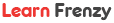• Quantitative Aptitude
• Data Interpretation
• Logical Reasoning
• Verbal Reasoning
• Non Verbal Reasoning
• Verbal Ability
• C Programming
• C++ Programming
• Java Programming
• Placement Papers
• HR Interview
• Technical Interview
• Group Discussion
• Submit Your Placement Paper & Experience
• General Online Tests
• Programming Online Tests
• Engineering Online Tests
• Engineering
• General Knowledge
• Exam Central
• Technical Online Tests
• Introduction

## Arithmetic Reasoning

Arithmetic reasoning: introduction.

Arithmetic reasoning involves the basic mathematical and arithmetic problems.

Arithmetic Reasoning is a part of mathematics which deals with the number sequence, mathematical operators, ratio and proportion, percentage, power and roots, sets and probability. The application of arithmetic reasoning is found in our day to day life activity like calculating the total amount of expenditure, a percentage of monthly income, to find the area of a land etc. The common arithmetic operators we use in problem are +,-,*,/, =.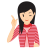Arithmetic reasoning test is designed to test the ability of a candidate to solve the various Mathematical problems which are encountered in day to day life. To solve the problems on Arithmetical reasoning, a candidate should have a knowledge of concepts of arithmetic or basic Mathematics.

Examples given below, will give you a better idea about the types of questions asked in various examinations.

Example 1.  If cost of 15 eggs is ₹75, then find out the cost of 4 dozens eggs.

(a) ₹240     (b) ₹300     (c) ₹150     (d) ₹185

Example 2.  If a number is multiplied by three-fourth of itself, then the value thus obtained is 10800. What is that number?

(a) ₹210            (b) ₹180

(c) ₹120            (d) ₹160

Solution: (c) Let the number be x.

According to the question,

x x ( x x 3/4) = 10800

Example 3.  The difference of the ages of Rohit and Axar is 12 yr. The ratio of their ages is 3 : 5. The age of Axar is

(a) 32 yr            (b) 24 yr

(c) 28 yr            (d) 30 yr

Solution: (d) Let present ages of Rohit and Axar be 3x and 5x, respectively.

According to the question, 5x - 3x = 12

Example 4.   There are deer and peacocks in a zoo. By counting heads they are 80. The number of their legs is 200. How many peacocks are there?

(a) 60            (b) 50

(c) 20            (d) 30

Solution: (a) Assuming deer = d and peacock = p                                                 x4 Head ------> d + p = 80  ---------------> 4d + 4p = 320  ....(i)

Legs ------> 4d + 2p = 200 -----------> 4d + 2p = 200  ....(ii)

From subtraction Eq. (ii) from Eq. (i), we get

Example 5.  In an examination, 78% of the total students who appeared were successful. If the total number of failures was 176 and 34% got first class, then how many students got first class?

(a) 272            (b) 112          (c) 210            (d) 254

Solution: (a) Lets total students appeared in an exam = y

Example 6.  At the end of a business conference all the ten people present, shake hands with each other only once. How many handshakes were there altogether?

(a) 20            (b) 45          (c) 55            (d) 90

Solution: (b) Total number of handshakes = n(n-1)/2

Where, n = number of people

Total number of handshakes

= 10 (10 - 1)/2

## Test-Taking Strategies

During a timed test, our inclination is to rush through each problem afraid that we’ll run out of time. Doing that in this particular part of the exam can be a recipe for disaster. Word problems can be tricky, so you have to carefully read each to discern exactly what is being asked for.

#2:  Determine the question

After reading the problem, the next step is to determine exactly what the question is asking.

#3:  Determine how to answer that question

In this step, you’ll need to look back at the problem and determine the best way to answer the question it is asking. This step will involve extracting the relevant facts from the problem.

#4:  Create an equation or equations

Once you have determined the best way to provide the answer and have gathered the relevant facts, you need to put those facts into an equation that will give you the correct answer.

#5:  Solve and review

In this final step, you will solve your equation or equations, do a quick review to make sure you have arrived at an answer that satisfies what the question is looking for and then record you answer.

LearnFrenzy provides you lots of fully solved Arithmetic Reasoning Questions and Answers with explanation.

• Arithmetic Reasoning - Base Level
• Arithmetic Reasoning - Expert Level#### IMAGES

1. how to solve arithmetic reasoning problems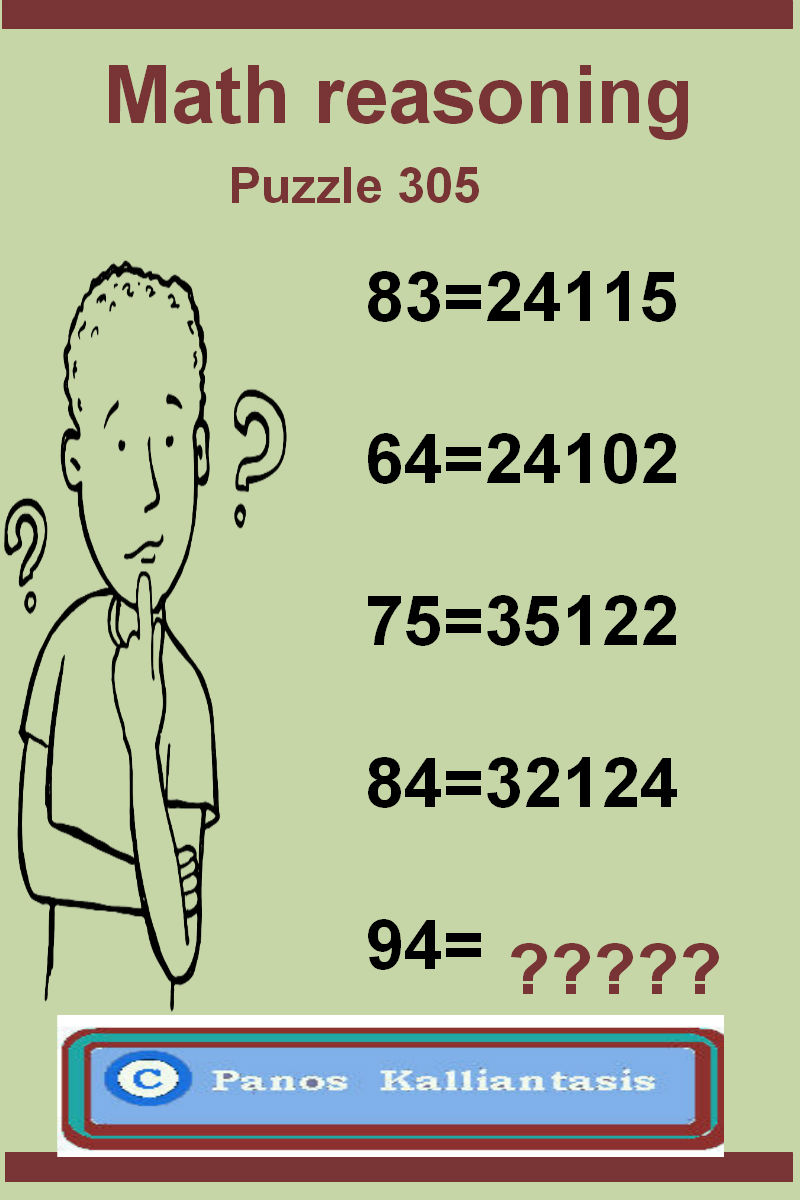2. How To Pass The Arithmetic Reasoning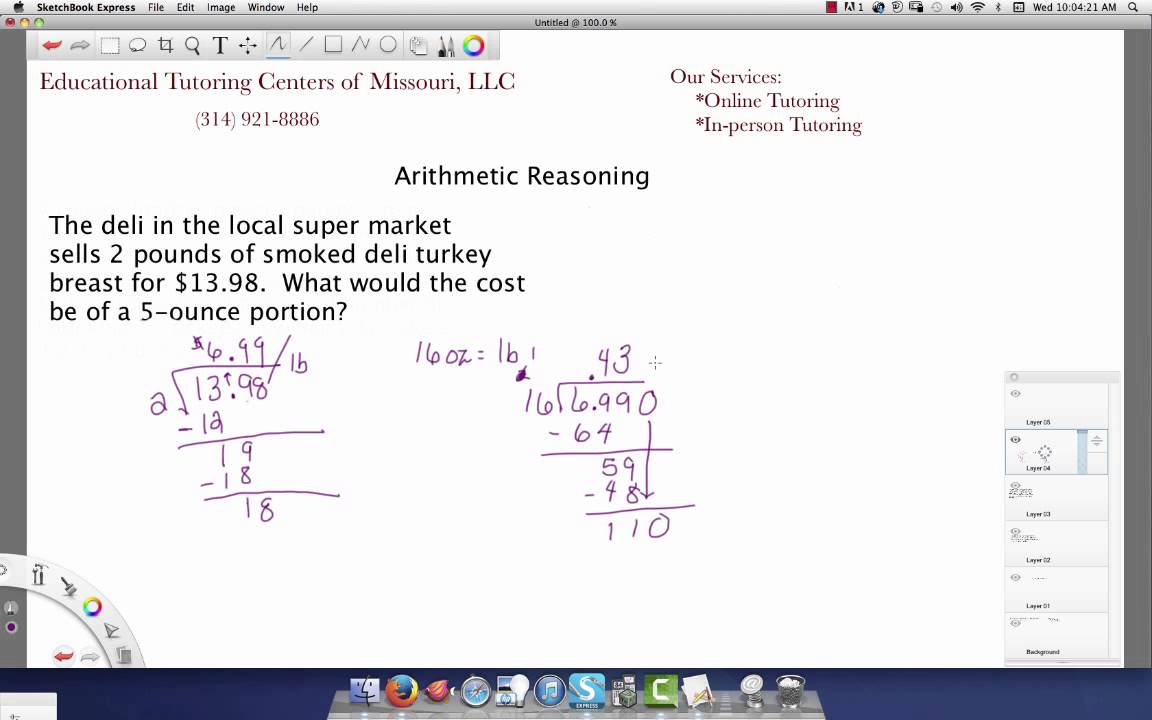3. Arithmetic Reasoning Test Solved & Explained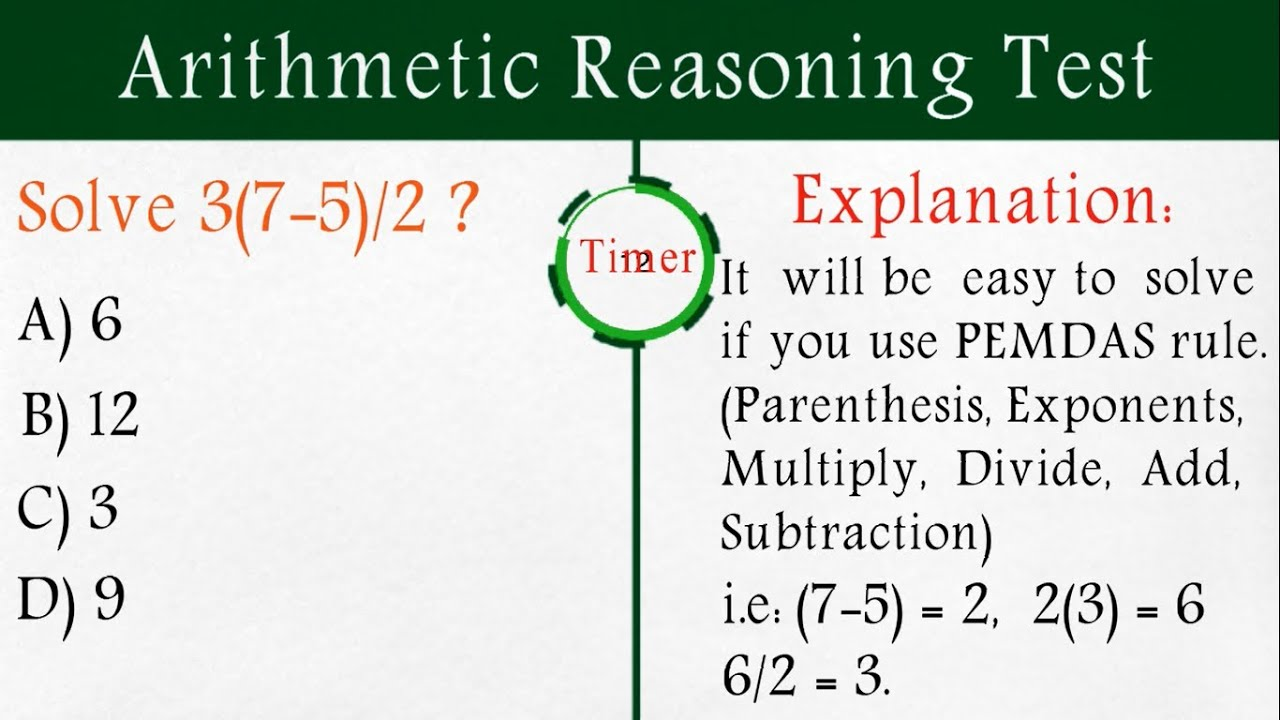4. Can You Solve This ASVAB Arithmetic Reasoning Word Problem?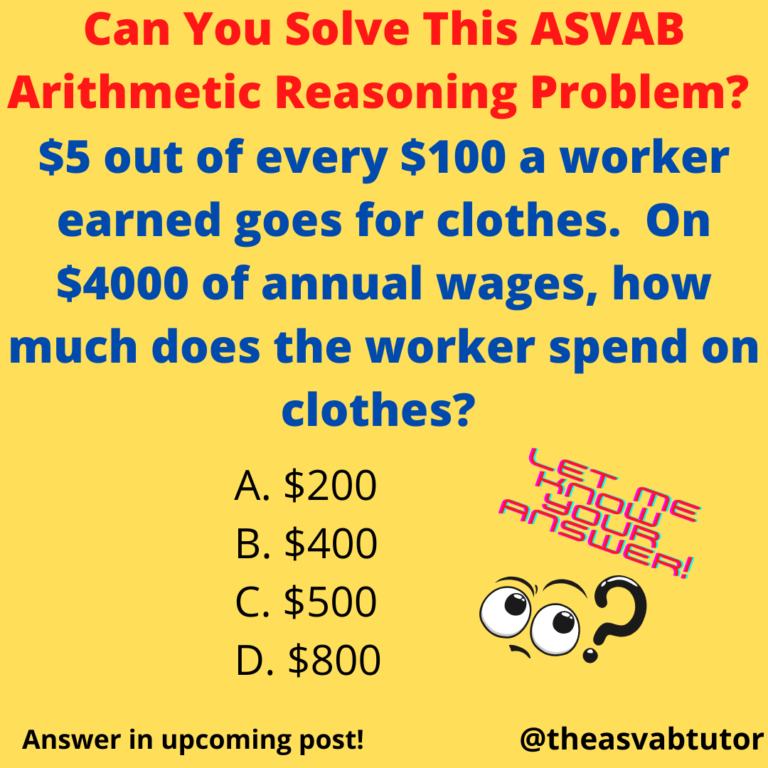5. how to solve arithmetic reasoning problems6. Brain teasers and puzzles: Math reasoning-puzzle 303#### VIDEO

1. How To Solve Arithmetic Sequence #shorts #arithmeticsequence #math #mathematics

2. Reasoning : Number Problem #ReasoningTimesShorts

3. Arithmetic and reasoning Numbers Topic #rrb #mathestricks

4. Mathematical Reasoning #reasoningquestions #Shorts

5. maths reasoning questions please find the question ❓❓❓#reasoning #mathsquestions solve in systemmm 😎

6. How to solve arithmetic progression

1. What Is Meant by Reasonableness in Math?

In math, reasonableness refers to the results of a calculation or problem-solving operation reflecting what is reasonable within the context of the given factors or values. Another method of establishing the reasonableness of an answer is t...

2. What Are the Six Steps of Problem Solving?

The six steps of problem solving involve problem definition, problem analysis, developing possible solutions, selecting a solution, implementing the solution and evaluating the outcome. Problem solving models are used to address issues that...

3. How to Solve Common Maytag Washer Problems

Maytag washers are reliable and durable machines, but like any appliance, they can experience problems from time to time. Fortunately, many of the most common issues can be solved quickly and easily. Here’s a look at how to troubleshoot som...

4. How to Solve ASVAB Arithmetic Reasoning Word Problems

Solving ASVAB math word problems isn't easy if you don't have a plan. In this video, we'll go over an ASVAB Arithmetic Reasoning Word

5. How to Do Arithmetic Reasoning : Math Concepts

ASVAB Math - How to Solve ASVAB Arithmetic Reasoning Word Problems: 3 Easy Steps with Practice! Duran Learning•26K views · 15:04 · Go to channel

6. Arithmetic Reasoning: Concepts, Tips, Formulas & Solved Examples

Arithmetic Reasoning Formulas · Addition: a/b + c/d = (ad + bc) / bd · Subtraction: a/b - c/d = (ad - bc) / bd · Multiplication: (a/b) * (c/d) = (a

7. Arithmetic Reasoning Solved Examples

Arithmetic Reasoning: Solved Examples · In a college, there are 34 girls who have car. If these constitute one fifth of the girls and the total no. · Joseph is

8. A 2023 Complete ASVAB Arithmetic Reasoning Study Guide

Steps to solving ASVAB word problems · Carefully read the problem · Determine the method used to answer · Setup the equations · Solve equations and review results.

9. ASVAB Arithmetic Reasoning Test Study Guide

Arithmetic reasoning refers to the process of solving math word problems – you know those questions you had in elementary, middle and high school that might

10. Page 1 of the Arithmetic Reasoning Study Guide for the ASVAB

solve the equation. As always, whenever you are uncertain about a specific

11. ASVAB Arithmetic Reasoning Practice ...

Arithmetic Reasoning practice test! Start Test · ASVAB

12. ASVAB Arithmetic Reasoning Practice Test

These questions are word problems that test your ability to use mathematics for thinking, reasoning, and problem solving. Our Arithmetic Reasoning practice

13. ASVAB Arithmetic Reasoning Math Study Guide

Click here to see example problems for each topic. You can practice and grow in each of these ASVAB Arithmetic Reasoning topics using the ASVAB

14. Arithmetic Reasoning

The common arithmetic operators we use in problem are +,-,*,/, =. MIND IT ! Arithmetic reasoning test is designed to test the ability of a candidate to solve